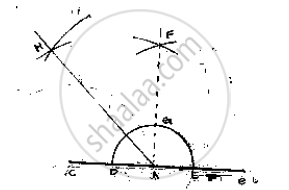# Construct the Angle of the Measurement: 1.135° - Mathematics

Construct the angle of the measurement:

1. 135°

#### SolutionSteps of construction:
1. Draw a line segment AB and produce BA to pint C
2. With center A and any radius, draw an arc which intersect AC to D and AB at E.

3. With center D and E and radius more than half of DE, draw two arcs which intersects
each other at F.
4. Join FA which intersect the arc in (2) at G
5. With center G and D radius more than 1/2GD,  draw two arcs which intersect each other at H
6. Join HA

∠ HAB = 135°

Concept: Basic Constructions
Is there an error in this question or solution?

#### APPEARS IN

RD Sharma Mathematics for Class 9
Chapter 16 Constructions
Exercise 16.2 | Q 11.4 | Page 9

Share